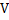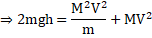# A gun of massfires a shell of masshorizontally and the energy of explosion is such as would be sufficient to project the shell vertically to a heightThe recoil velocity of the gun is a)b)c)d)## Question ID - 100194 :- A gun of massfires a shell of masshorizontally and the energy of explosion is such as would be sufficient to project the shell vertically to a heightThe recoil velocity of the gun is a)b)c)d)3537

(a)

Lis the energy of explosion, then since it is just sufficient to carry the bullet to a heightsoWe haveNow, ifandare the respective velocities of the bullet and the gun, respectively, then, from the law of conservation of linear momentum,( i )

Also,[from Eq. (i)]Next Question :

Figure shows a thin metallic triangular sheetThe mass of the sheet is. The moment of inertia of the sheet about sideisa)b)c)d)